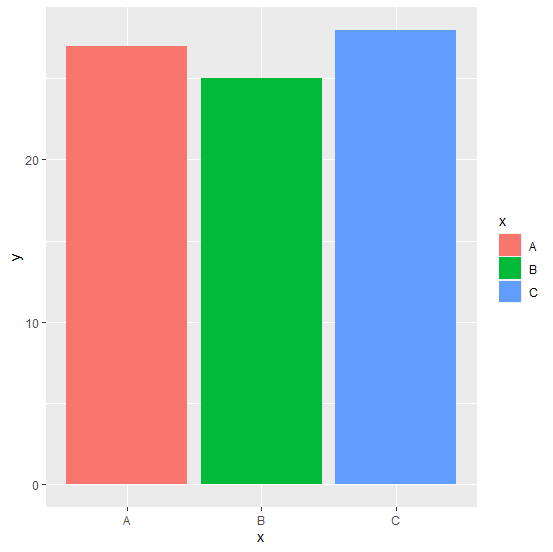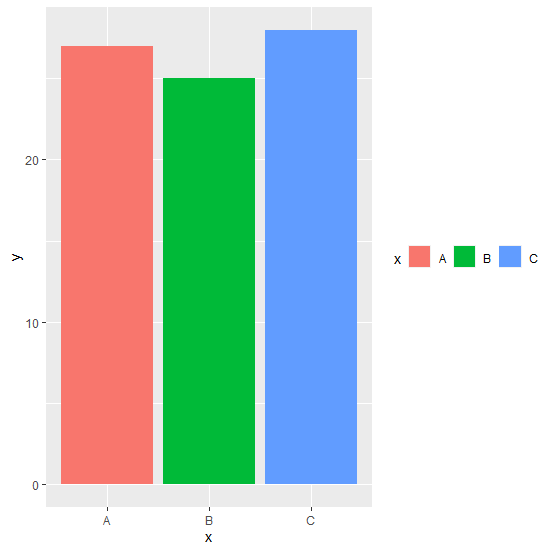# How to create horizontal legend using ggplot2 in R?

The default legend direction is vertical but it can be changed to horizontal as well and for this purpose we can use legend.direction argument of theme function of ggplot2 package. For example, if we want to create a bar chart with x as categories and y as frequencies tjat are contained in a data frame df then the bar chart with horizontal legends for categories in x can be created as −

ggplot(df,aes(x,y,fill=x))+geom_bar(stat="identity")+theme(legend.direction="horizontal")

## Example

Consider the below data frame −

Live Demo

> x<-c("A","B","C")
> y<-c(27,25,28)
> df<-data.frame(x,y)
> df

## Output

x y
1 A 27
2 B 25
3 C 28

## Example

> library(ggplot2)
> ggplot(df,aes(x,y,fill=x))+geom_bar(stat="identity")

## OutputCreating the chart with horizontal legend −

## Example

> ggplot(df,aes(x,y,fill=x))+geom_bar(stat="identity")+theme(legend.direction="horizontal")

## Output